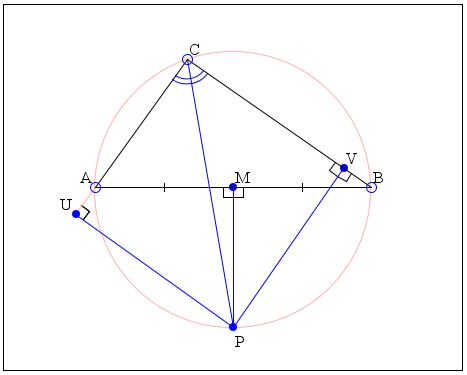# All Triangles Are IsoscelesHow Is It Possible?

16 February 2016, Created with GeoGebra

I plan to show that all triangles are isosceles, or at least all acute triangles are. Given such an acute ΔABC, find the intersection P of the bisector of angle C and the perpendicular bisector at the midpoint M of side AB. Drop perpendiculars PU and PV on the sides AC and BC, respectively.

Now, since MP is the perpendicular bisector of AB, triangles AMP and BMP are equal by SAS. Therefore, AP = BP. Right triangles CPU and CPV share a side (CP) and have equal angles at C. By ASA, they are equal. Therefore, CU = CV and PU = PV. In right triangles APU and BPV,

PU = PV and
AP = BP.

They are therefore equal by SSS. Therefore, AU = BV. But then

AC = AU + CU = BV + CV = BC.

Note that we showed that any two sides of a triangle are equal. As a consquence, we may rightly conclude that all three are, meaning that all triangles are equilateral.

How come?The problem is with the drawing. The angle bisector of C and the perpendicular bisector of AB always intersect on the circumcircle of ΔABC. This is because the angle bisector from C divides the arc AB opposite C into two equal parts. The same effect is obviously achieved by the perpendicular bisector of AB that passes through the circumcenter.

The original diagram is intentionally misleading.

### References

1. V. M. Bradis et al, Lapses in Mathematical Reasoning, Dover, 1999, pp. 137-138
2. C. W. Dodge, Euclidean Geometry and Transformations, Dover, 2004 (reprint of 1972 edition), 7.23.•